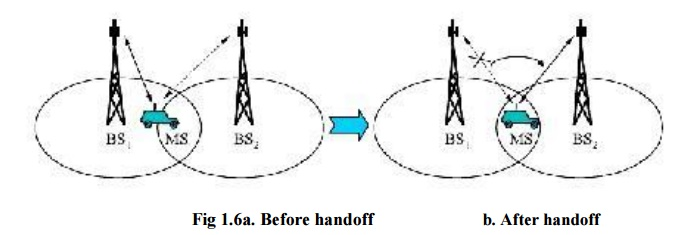Home | | Mobile and Pervasive Computing | Fundamental Concept In Cellular Technology

# Fundamental Concept In Cellular Technology

The radio spectrum contains many bands that are allocated and used for commercial, personal, and military applications.

FUNDAMENTAL CONCEPT IN CELLULAR TECHNOLOGY

The radio spectrum contains many bands that are allocated and used for commercial, personal, and military applications. Fifty (50) MHz of spectrum allocated to cellular networks exists in the 824-849 MHz and the 869-894 MHz bands (Pagett, 1995). These bands are then further subdivided into 832 channels allowing many users in the same area to simultaneously access the network. Types of cellular network access are:

 Advanced mobile phone system (AMPS).

 Time division multiple access (TDMA).

 Code division multiple access (CDMA).

Cells are combined together to form clusters. There are 2 types of formation of clusters

·        Three cells forming a cluster.

·        Seven cells forming a cluster.The set of frequencies can be reused in other cells, provided that the same frequencies are not reused in adjacent nearby cells as that would cause co-channel interference. So never use same frequencies at same time with in the interference range.Sectorized antenna is an another method to reduce the interference.

Channel Assignment Strategies:

Fixed frequency assignment.

Dynamic frequency assignment.

Fixed frequency assignment:

If certain frequency are assigned to certain cells then it is called fixed channel allocation (FCA). In fixed channel assignment strategy each cell is allocated a fixed number of voice channels. The problem related is dissimilar traffic load occurs in dissimilar cell.

Dynamic frequency assignment:

If the frequency is borrowed and assigned to cells then it is called dynamic channel allocation(DCA). In dynamic channel assignment strategy channels are temporarily assigned for use in cells for the duration of the call. As the frequencies are recurring, the transmission power is restricted to stay away from interference with subsequent cell using the same frequency.

If the cell has heavy traffic and its neighboring cell has less load then the frequencies can be borrowed and assigned to the cell having heavy load. This is called borrowing channel allocation(BCA).The idea of breathe is that the cell can cover bulky area under light load and its size shrink under heavy load.CDM system faces a problem of cell size depending upon load.

Handoff process:

Handoff is an important task in cellular network. when a MS moves into another cell, while the conversation is still in progress, the MSC automatically transfers the call to a new FDD channel without disturbing the conversation. This process is called as handoff.Handoff performance metrics:

 The probability of a new call being blocked is referred as call blocking Probability.

 The probability that the call is ended due to handoff is the call dropping probability.

 The probability that a admitted call is not dropped before ended is the call completion probability.

 The probability that a handoff is executed while the response conditions are inadequate is referred as Probability of unsuccessful handoff.

 The probability that a handoff cannot be effectively completed is the handoff blocking probability.

 The probability that a handoff occur earlier than call termination is the handoff probability.

       The number of handoffs per unit time is referred as rate of handoff.

       The duration of moment during a handoff in which a mobile is not connected to either base station is the Interruption duration.

       Distance the mobile moves from the point at which the handoff should occur to the point at which it does occur is the handoff delay.

Handoff strategy used to determine instant of handoff:

Relative signal strength

Prediction techniques

Relative signal strength with hysteresis and threshold

Relative signal strength with hysteresis

Relative signal strength with threshold

Frequency reuse:

Frequency reuse, or, frequency planning, is a technique of reusing frequencies and channels within a communication system to improve capacity and spectral efficiency.

The characteristic of a cellular network is the ability to re-use frequencies to increase both coverage and capacity. Adjacent cells must use different frequencies to avoid interference, however there is no difficult with two cells sufficiently far apart in use on the same frequency. 10 to 50 frequencies are assigned to each cell. The elements that determine frequency reuse are the reuse distance and the reuse factor. The reuse distance, D is calculated asN- number of cells per cluster.

The rate at which the same frequency can be used in the network is called frequency reuse factor. It is 1/K

K - Number of cells which cannot use the same frequencies for transmission.

Common values for frequency reuse factor are 1/3, 1/4, 1/7, 1/9 and 1/12.Factors limiting Frequency reuse are co-channel interference and adjacent channel interference.Each Colour/letter uses the same frequency band.

Study Material, Lecturing Notes, Assignment, Reference, Wiki description explanation, brief detail
Mobile Networks : Cellular Wireless Network : Fundamental Concept In Cellular Technology |